Want to share your content on R-bloggers? click here if you have a blog, or here if you don't.

# Introduction

Survival analysis is used to analyze the time until the occurrence of an event (or multiple events). Cox models—which are often referred to as semiparametric because they do not assume any particular baseline survival distribution—are perhaps the most widely used technique; however, Cox models are not without limitations and parametric approaches can be advantageous in many contexts. For instance, parametric survival models are essential for extrapolating survival outcomes beyond the available follow-up data. For this reason they are nearly always used in health-economic evaluations where it is necessary to consider the lifetime health effects (and costs) of medical interventions.

R contains a large number of packages related to biostatistics and its support for parametric survival modeling is no different. Below we will examine a range of parametric survival distributions, their specifications in R, and the hazard shapes they support. We will then show how the flexsurv package can make parametric regression modeling of survival data straightforward.

# Survival distributions

The primary quantity of interest in survival analysis is the survivor function, defined as the probability of survival beyond time $t$,

where $T$ is a random variable denoting the time that the event occurs. The survival function is the complement of the cumulative density function (CDF), $F(t) = \int_0^t f(u)du$, where $f(t)$ is the probability density function (PDF).

The hazard function, or the instantaneous rate at which an event occurs at time $t$ given survival until time $t$ is given by,

The survivor function can also be expressed in terms of the cumulative hazard function, $\Lambda(t) = \int_0^t \lambda (u)du$,

R functions for parametric distributions used for survival analysis are shown in the table below. The default stats package contains functions for the PDF, the CDF, and random number generation for many of the distributions. Additional distributions as well as support for hazard functions are provided by flexsurv.

PDF CDF Hazard Random sampling
Exponential stats::dexp stats::pexp flexsurv::hexp flexsurv::rexp
Weibull (AFT) stats::dweibull stats::pweibull flexsurv::hweibull stats::rweibull
Weibull (PH) flexsurv::dweibullPH flexsurv::pweibullPH flexsurv::hweibullPH flexsurv::rweibullPH
Gompertz flexsurv::dgompertz flexsurv::pgompertz flexsurv::hgompertz flexsurv::rgompertz
Gamma stats::dgamma stats::pgamma flexsurv::hgamma stats::rgamma
Lognormal stats::dlnorm stats::plnorm flexsurv::hlnorm stats::rlnorm
Log-logistic flexsurv::dllogis flexsurv::pllogis flexsurv::hllogis flexsurv::rllogis
Generalized gamma flexsurv::dgengamma flexsurv::pgengamma flexsurv::hgengamma flexsurv::rgengamma

The parameterizations of these distributions in R are shown in the next table. The parameter of primary interest (in flexsurv) is colored in red—it is known as the location parameter and typically governs the mean or location for each distribution. The other parameters are ancillary parameters that determine the shape, variance, or higher moments of the distribution. These parameters impact the hazard function, which can take a variety of shapes depending on the distribution:

• the exponential distribution only supports a constant hazard;
• the Weibull, Gompertz, and gamma distributions support monotonically increasing and decreasing hazards;
• the log-logistic and lognormal distributions support arc-shaped and monotonically decreasing hazards; and
• the generalized gamma distribution supports an arc-shaped, bathtub-shaped, monotonically increasing, and monotonically decreasing hazards.
PDF CDF Hazard Parameters Hazard shape
Exponential $\lambda e^{-\lambda t}$ $1 – e^{-\lambda t}$ $\lambda$ $\color{red}{\text{rate}} = \lambda \gt 0$ Constant
Weibull (AFT) $\frac{a}{b}\left(\frac{t}{b}\right)^{a-1}e^{-(t/b)^a}$ $1 – e^{-(t/b)^a}$ $\frac{a}{b}\left(\frac{t}{b}\right)^{a-1}$ $\text{shape} = a \gt 0 \\ \color{red}{\text{scale}} = b \gt 0$ Constant, monotonically increasing/decreasing
Weibull (PH)a $a m t^{a-1} e^{-m t^a}$ $1 – e^{-mt^a}$ $amt^{a-1}$ $\text{shape} = a \gt 0 \\ \color{red}{\text{scale}} = m \gt 0$ Constant, monotonically increasing/decreasing
Gompertz $b e^{at} \exp\left[-\frac{b}{a}(e^{at}-1)\right]$ $1 – \exp\left[-\frac{b}{a}(e^{at}-1)\right]$ $b e^{at}$ $\text{shape} = a \in (-\infty, \infty) \\ \color{red}{\text{rate}} = b \gt 0$ Constant, monotonically increasing/decreasing
Gammab $\frac{b^a}{\Gamma(a)}t^{a -1}e^{-bt}$ $\frac{\gamma(a, bt)}{\Gamma(a)}$ $f(t)/S(t)$ $\text{shape} = a \gt 0 \\ \color{red}{\text{rate}} = b \gt 0$ Constant, monotonically increasing/decreasing
Lognormal $\frac{1}{t\sigma\sqrt{2\pi}}e^{-\frac{(\ln t – \mu)^2}{2\sigma^2}}$ $\Phi\left(\frac{\ln t – \mu}{\sigma}\right)$ $f(t)/S(t)$ $\color{red}{\text{meanlog}} = \mu \in (-\infty, \infty) \\ \text{sdlog} = \sigma \gt 0$ Arc-shaped, monotonically decreasing
Log-logistic $\frac{(a/b)(t/b)^{a-1}}{\left(1 + (t/b)^a\right)^2}$ $\frac{1}{(1+(t/b)^a)}$ $1-\frac{(a/b)(t/b)^{a-1}}{\left(1 + (t/b)^a\right)}$ $\text{shape} = a \gt 0 \\ \color{red}{\text{scale}} = b \gt 0$ Arc-shaped, monotonically decreasing
Generalized gammac $\frac{|Q|(Q^{-2})^{Q^{-2}}}{\sigma t \Gamma(Q^{-2})} \exp\left[Q^{-2}\left(Qw-e^{Qw}\right)\right]$ $\begin{cases} \frac{\gamma(Q^{-2}, u)}{\Gamma(Q^{-2})} \text{ if } Q \neq 0 \\ \Phi(w) \text{ if } Q = 0 \end{cases}$ $f(t)/S(t)$ $\color{red}{\text{mu}} = \mu \in (-\infty, \infty) \\ \text{sigma} = \sigma \gt 0 \\ \text{Q} = Q \in (-\infty, \infty)$ Arc-shaped, bathtub-shaped, monotonically increasing/decreasing
a The proportional hazard (PH) model is a reparameterization of the accelerated failure time (AFT) model with $m = b^{-a}$.
b $\Gamma(z) = \int_{0}^{\infty} x^{z-1}e^{-x} dx$ is the gamma function and $\gamma(s,x) = \int_{0}^{x} t^{s-1}e^{-t}dt$ is the lower incomplete gamma function.
c $w = (log(t) – \mu)/\sigma; u = Q^{-2}e^{Qw}$.

# Shapes of hazard functions

We will now examine the shapes of the hazards in a bit more detail and show how both the location and shape vary with the parameters of each distribution. Readers interested in a more interactive experience can also view my Shiny app here.

To do so we will load some needed packages: we will use flexsurv to compute the hazards, data.table as a fast alternative to data.frame, and ggplot2 for plotting.

library("flexsurv")
library("data.table")
library("ggplot2")
ggplot2::theme_set(theme_minimal())


We can create a general function for computing hazards for any general hazard function given combinations of parameter values at different time points. The key to the function is mapply, a multivariate version of sapply. To illustrate, let’s compute the hazard from a Weibull distribution given 3 values each of the shape and scale parameters at time points 1 and 2. The output is a matrix where each row corresponds to a time point and each column is combination of the shape and scale parameters. For example, the second row and third column is the hazard at time point 2 given a shape parameter of 1.5 and a scale parameter of 1.75.

mapply(flexsurv::hweibull,
shape = c(.5, 1, 1.5),
scale = c(.25, 1, 1.75),
MoreArgs = list(x = 1:2))

##           [,1] [,2]      [,3]
## [1,] 1.0000000    1 0.6479391
## [2,] 0.7071068    1 0.9163243


The more general function uses mapply to return a data.table of hazards at all possible combinations of the parameter values and time points. Factor variables and intuitive names are also returned to facilitate plotting with ggplot2.

hazfun <- function(FUN, param_vals, times){
# Compute hazard for all possible combinations of parameters and times
values <- do.call("mapply", c(list(FUN = FUN),
as.list(expand.grid(param_vals)),
list(MoreArgs = list(x = times))))
x <- data.table(expand.grid(c(param_vals, list(time = times))),
value = c(t(values)))

# Create factor variables and intuitive names for plotting
param_names <- names(param_vals)
x[[param_names]] <- factor(x[[param_names]],
levels = unique(x[[param_names]]))
if (length(param_vals) > 1){
for (j in 2:length(param_vals)){
ordered_vals <- unique(x[[param_names[j]]])
x[[param_names[j]]] <- paste0(param_names[j], " = ", x[[param_names[j]]])
factor_levels <- paste0(param_names[j], " = ", ordered_vals)
x[[param_names[j]]] <- factor(x[[param_names[j]]],
levels = factor_levels)
}
}

# Return
return(x)
}


## Exponential distribution

The exponential distribution is parameterized by a single rate parameter and only supports a hazard that is constant over time. The hazard is simply equal to the rate parameter.

times <- seq(1, 10, 1)
rate <- seq(1, 5, 1)
haz_exp <- hazfun(flexsurv::hexp,
list(rate = rate),
times = times)
ggplot(haz_exp, aes(x = time, y = value, col = rate)) +
geom_line() + xlab("Time") + ylab("Hazard") +
scale_y_continuous(breaks = rate)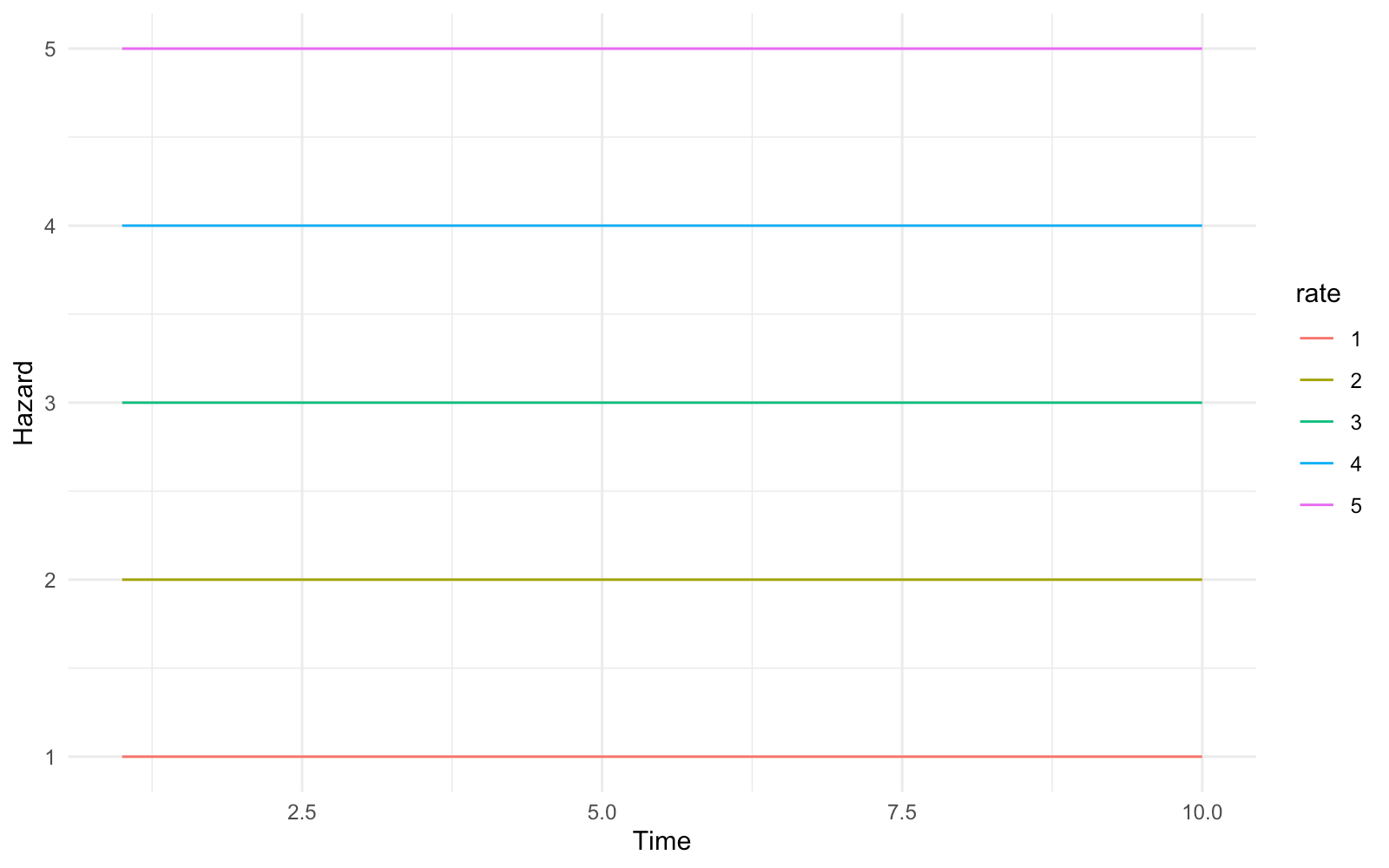## Weibull distribution (AFT)

The Weibull distribution can be parameterized as both an accelerated failure time (AFT) model or as a proportional hazards (PH) model. The parameterization in the base stats package is an AFT model.

The hazard is decreasing for shape parameter $a < 1$ and increasing for $a > 1$. For $a = 1$, the Weibull distribution is equivalent to an exponential distribution with rate parameter $1/b$ where $b$ is the scale parameter.

wei_shape <- seq(.25, 3, .25)
haz_wei <- hazfun(flexsurv::hweibull,
list(scale = seq(2, 5, .5),
shape = wei_shape),
times = times)
ggplot(haz_wei, aes(x = time, y = value, col = scale)) +
geom_line() + facet_wrap(~shape, scales = "free_y") +
xlab("Time") + ylab("Hazard")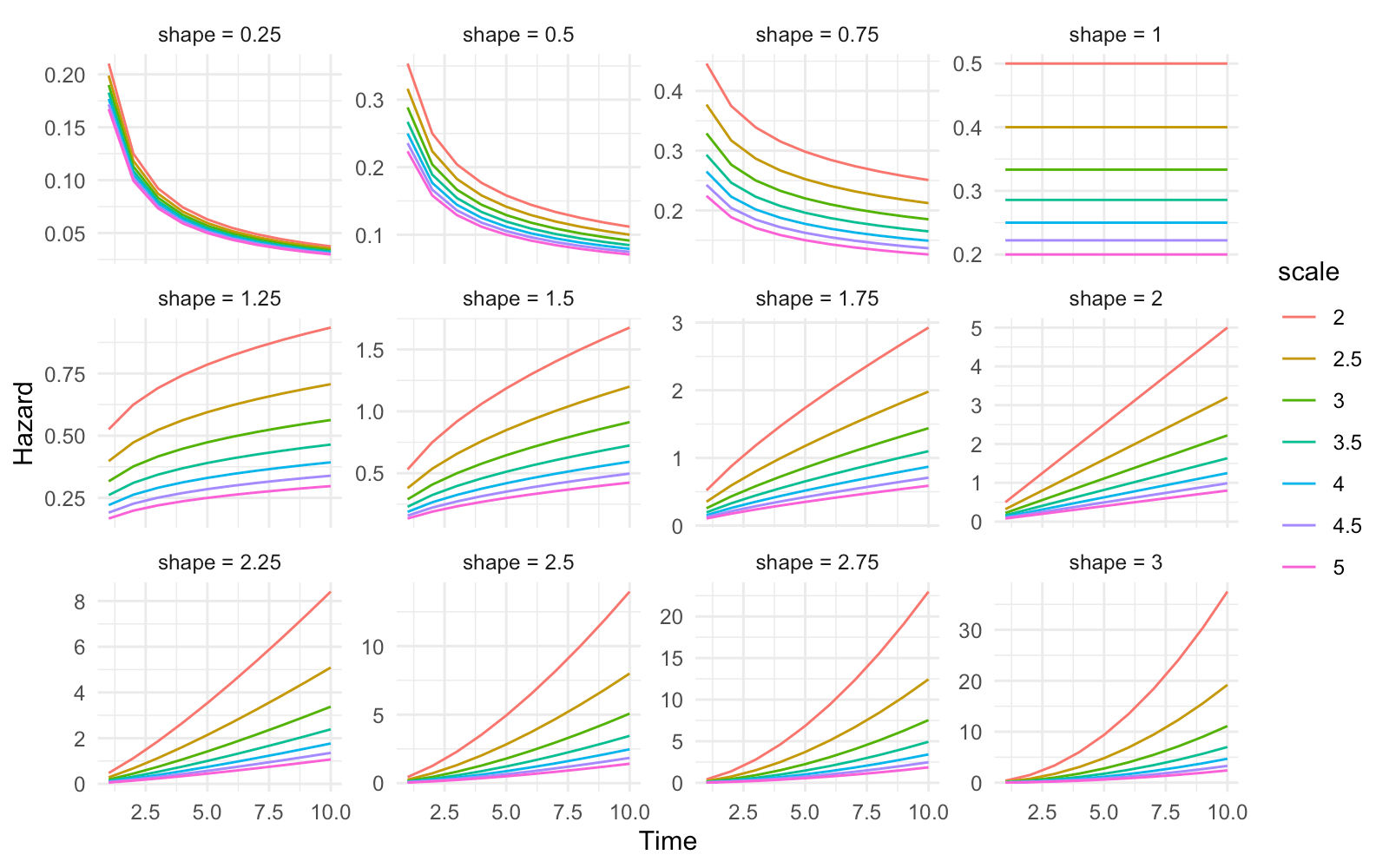## Weibull distribution (PH)

flexsurv provides an alternative PH parameterization of the Weibull model with the same shape parameter $a$ and a scale parameter $m = b^{-a}$ where $b$ is the scale parameter in the AFT model.

The hazard is again decreasing for $a < 1$, constant for $a = 1$, and increasing for $a > 1$. Note that for $a = 1$, the PH Weibull distribution is equivalent to an exponential distribution with rate parameter $m$.

haz_weiPH <- hazfun(flexsurv::hweibullPH,
list(scale = seq(2, 5, .5),
shape = wei_shape),
times = times)
ggplot(haz_weiPH, aes(x = time, y = value, col = scale)) +
geom_line() + facet_wrap(~shape, scales = "free_y") +
xlab("Time") + ylab("Hazard")## Gompertz distribution

The Gompertz distribution is parameterized by a shape parameter $a$ and rate parameter $b$. The hazard is increasing for $a > 0$, constant for $a = 0$, and decreasing for $a < 0$. When $a = 0$, the Gompertz distribution is equivalent to an exponential distribution with rate parameter $b$.

haz_gomp <- hazfun(flexsurv::hgompertz,
list(rate = seq(.5, 3, .5),
shape = seq(-2, 2, .5)),
times = times)
ggplot(haz_gomp, aes(x = time, y = value, col = rate)) +
geom_line() + facet_wrap(~shape, scales = "free_y") +
xlab("Time") + ylab("Hazard")## Gamma distribution

The gamma distribution is parameterized by a shape parameter $a$ and a rate parameter $b$. Like the Weibull distribution, the hazard is decreasing for $a < 1$, constant for $a = 1$, and increasing for $a >1$. In the case where $a = 1$, the gamma distribution is an exponential distribution with rate parameter $b$.

haz_gam <- hazfun(flexsurv::hgamma,
list(rate = seq(.5, 3, .5),
shape = c(1e-5, .5, 1, seq(2, 6))),
times = times)
ggplot(haz_gam, aes(x = time, y = value, col = rate)) +
geom_line() + facet_wrap(~shape, scales = "free_y") +
xlab("Time") + ylab("Hazard")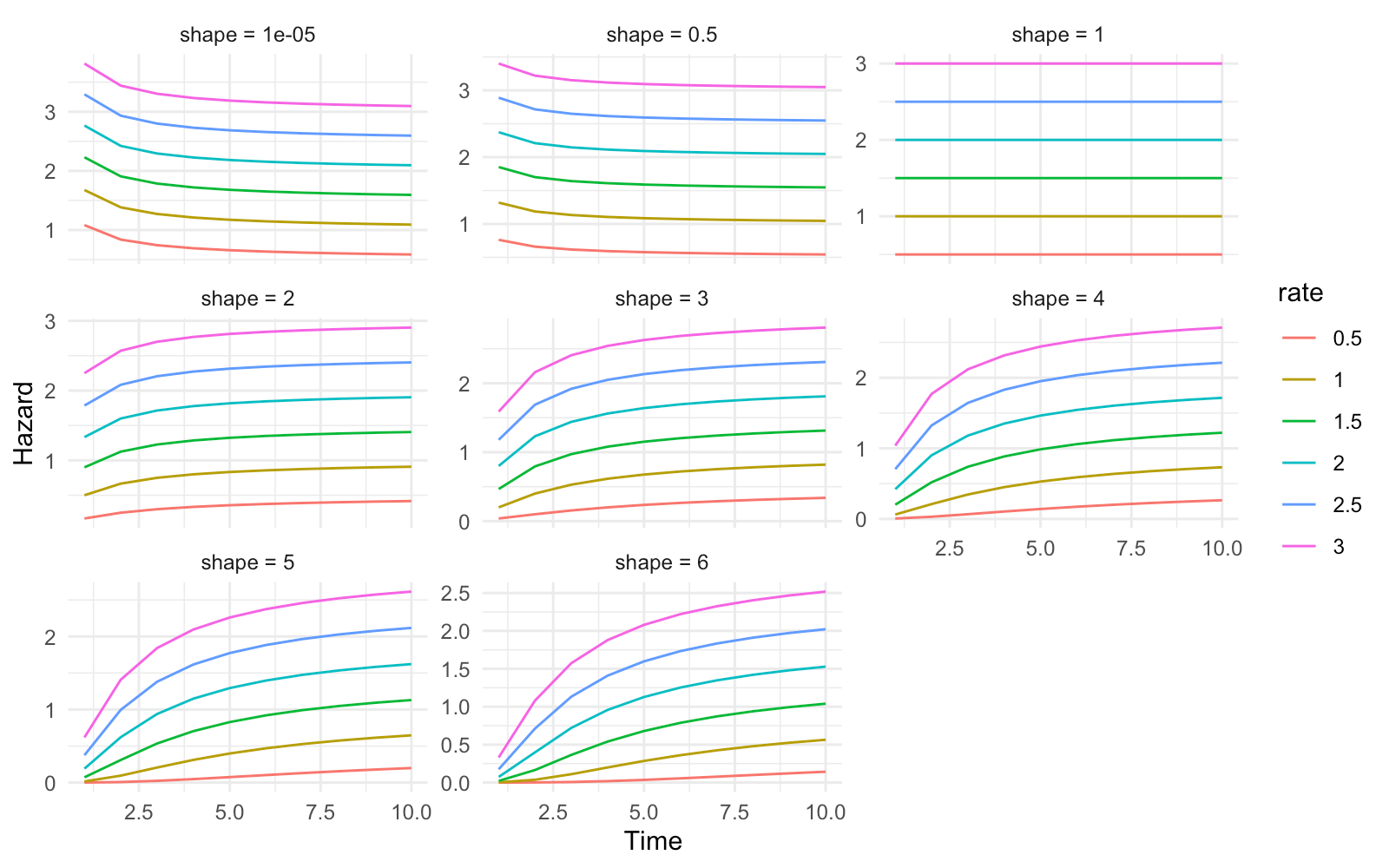## Lognormal distribution

The lognormal distribution is parameterized by the mean $\mu$ and standard deviation $\sigma$ of survival time on the log scale. The lognormal hazard is either monotonically decreasing or arc-shaped. Note that the shape of the hazard depends on the values of both $\mu$ and $\sigma$.

haz_lnorm <- hazfun(flexsurv::hlnorm,
list(meanlog = seq(0, 4, .5),
sdlog = seq(.5, 3, .5)),
times = seq(0, 20, .1))
ggplot(haz_lnorm, aes(x = time, y = value, col = meanlog)) +
geom_line() + facet_wrap(~sdlog, scales = "free_y") +
xlab("Time") + ylab("Hazard")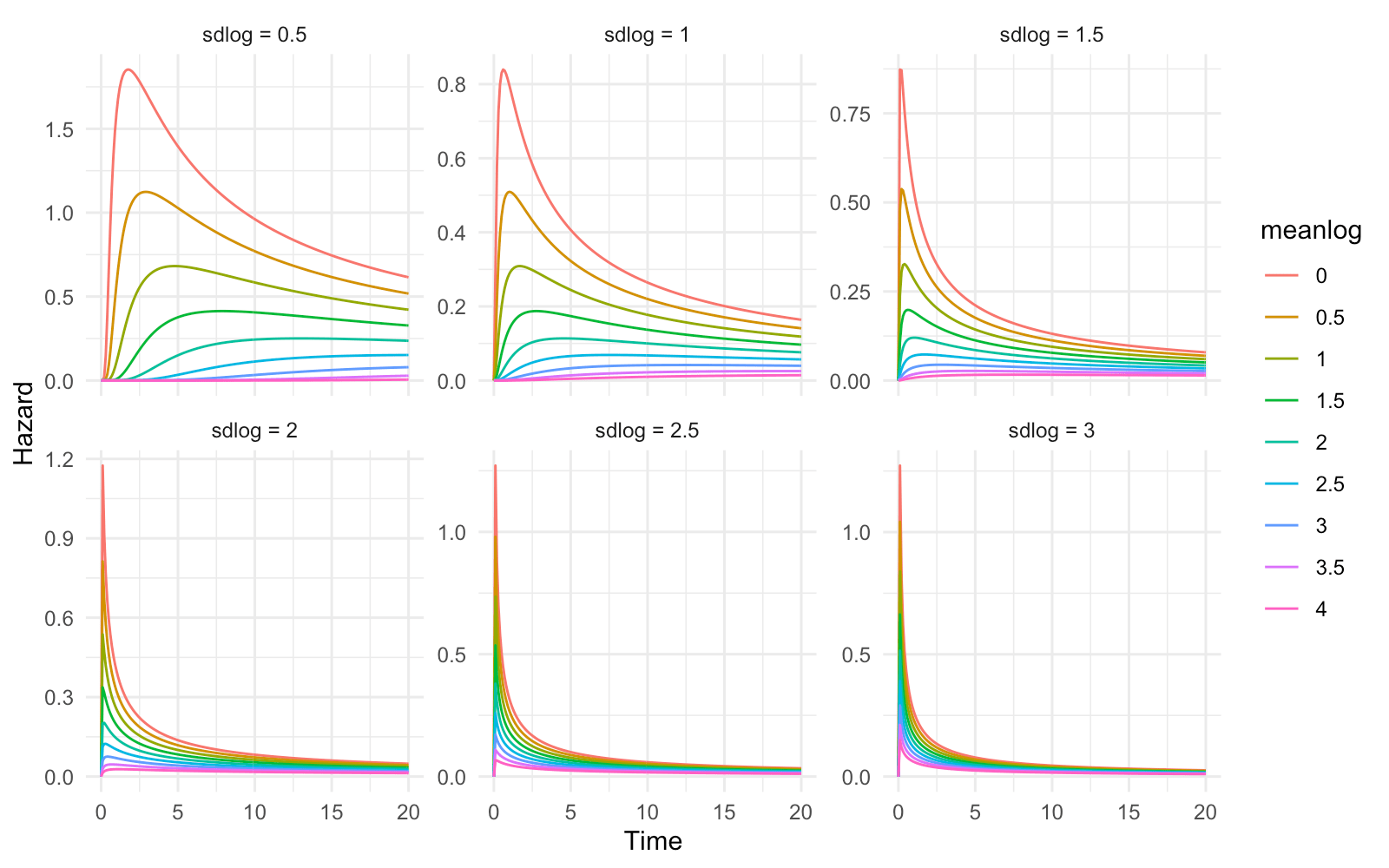## Log-logistic distribution

The log-logistic distribution is parameterized by a shape parameter $a$ and a scale parameter $b$. When $a > 1$, the hazard function is arc-shaped whereas when $a \leq 1$, the hazard function is decreasing monotonically.

haz_llogis <- hazfun(flexsurv::hllogis,
list(scale = seq(.5, 4, .5),
shape = seq(.5, 3, .5)),
times = seq(0, 20, 1))
ggplot(haz_llogis, aes(x = time, y = value, col = scale)) +
geom_line() + facet_wrap(~shape, scales = "free_y") +
xlab("Time") + ylab("Hazard")## Generalized gamma distribution

The generalized gamma distribution is parameterized by a location parameter $\mu$, a scale parameter $\sigma$, and a shape parameter $Q$. It is the most flexible distribution reviewed in this post and includes the exponential ($Q = \sigma = 1$), Weibull ($Q = 1$), gamma ($Q = \sigma$), and lognormal ($Q = 0$) distributions as special cases.

Each row in the figure corresponds to a unique value of $\sigma$ and each column corresponds to a unique value of $Q$.The generalized gamma distribution is quite flexible as it supports hazard functions that are monotonically increasing, monotonically decreasing, arc-shaped, and bathtub shaped.

haz_gengamma <- hazfun(flexsurv::hgengamma,
list(mu = seq(1, 4, .5),
sigma = seq(.5, 2, .5),
Q = seq(-3, 3, 1)),
times = seq(0, 30, 1))
ggplot(haz_gengamma, aes(x = time, y = value, col = mu)) +
geom_line() +
facet_wrap(sigma ~ Q,
ncol = length(levels(haz_gengamma$Q)), scales = "free") + xlab("Time") + ylab("Hazard") + theme(legend.position = "bottom") + guides(col = guide_legend(nrow = 2, byrow = TRUE))# Regression In flexsurv, survival models are fit to the data using maximum likelihood. Each parameter can be modeled as a function of covariates$z$, where$\alpha_l$is the$l$th parameter and$g^{-1}()$is a link function (typically$log()$if the parameter is strictly positive and the identity function if the parameter is defined on the real line). We will illustrate by modeling survival in a dataset of patients with advanced lung cancer from the survival package. The dataset uses a status indicator where 2 denotes death and 1 denotes alive at the time of last follow-up; we will convert this to the more traditional coding where 0 is dead and 1 is alive. dat <- data.table(survival::lung) dat[, status := ifelse(status == 1, 0, 1)] head(dat) ## inst time status age sex ph.ecog ph.karno pat.karno meal.cal wt.loss ## 1: 3 306 1 74 1 1 90 100 1175 NA ## 2: 3 455 1 68 1 0 90 90 1225 15 ## 3: 3 1010 0 56 1 0 90 90 NA 15 ## 4: 5 210 1 57 1 1 90 60 1150 11 ## 5: 1 883 1 60 1 0 100 90 NA 0 ## 6: 12 1022 0 74 1 1 50 80 513 0  ## Intercept only model We will begin by estimating intercept only parametric regression models (i.e., without covariates). But first, it’s helpful to estimate the hazard function (among all patients) using nonparametric techniques. We can do this using the kernel density estimator from the muhaz package. library("muhaz") kernel_haz_est <- muhaz(dat$time, dat$status) kernel_haz <- data.table(time = kernel_haz_est$est.grid,
est = kernel_haz_est$haz.est, method = "Kernel density")  Then we can use flexsurv to estimate intercept only models for a range of probability distributions. The hazard function for each fitted model is returned using summary.flexsurvreg(). dists <- c("exp", "weibull", "gompertz", "gamma", "lognormal", "llogis", "gengamma") dists_long <- c("Exponential", "Weibull (AFT)", "Gompertz", "Gamma", "Lognormal", "Log-logistic", "Generalized gamma") parametric_haz <- vector(mode = "list", length = length(dists)) for (i in 1:length(dists)){ fit <- flexsurvreg(Surv(time, status) ~ 1, data = dat, dist = dists[i]) parametric_haz[[i]] <- summary(fit, type = "hazard", ci = FALSE, tidy = TRUE) parametric_haz[[i]]$method <- dists_long[i]
}
parametric_haz <- rbindlist(parametric_haz)


We can plot the hazard functions from the parametric models and compare them to the kernel density estimate.

haz <- rbind(kernel_haz, parametric_haz)
haz[, method := factor(method,
levels = c("Kernel density",
dists_long))]
n_dists <- length(dists)
ggplot(haz, aes(x = time, y = est, col = method, linetype = method)) +
geom_line() +
xlab("Days") + ylab("Hazard") +
scale_colour_manual(name = "",
values = c("black", rainbow(n_dists))) +
scale_linetype_manual(name = "",
values = c(1, rep_len(2:6, n_dists)))The kernel density estimate is monotonically increasing and the slope increases considerably after around 500 days. The arc-shaped lognormal and log-logistic hazards and the constant exponential hazard do not fit the data well. The best performing models are those that support monotonically increasing hazards (Gompertz, Weibull, gamma, and generalized gamma). The flexible generalized gamma and the Gompertz models perform the best with the Gompertz modeling the increase in the slope of the hazard the most closely.

As mentioned above each parameter can be modeled as a function of covariates. By default, flexsurv only uses covariates to model the location parameter. To demonstrate, we will let the rate parameter of the Gompertz distribution depend on the ECOG performance score (0 = good, 5 = dead), which describes a patient’s level of functioning and has been shown to be a prognostic factor for survival. The model is fit using flexsurvreg().

dat[, ph.ecog := factor(ph.ecog)]

# Covariates on rate parameter only
fit_covs1 <- flexsurvreg(Surv(time, status) ~ ph.ecog,
data = dat, dist = "gompertz")
print(fit_covs1)

## Call:
## flexsurvreg(formula = Surv(time, status) ~ ph.ecog, data = dat,
##     dist = "gompertz")
##
## Estimates:
##           data mean  est        L95%       U95%       se         exp(est)
## shape            NA   0.001595   0.000925   0.002265   0.000342         NA
## rate             NA   0.001070   0.000725   0.001579   0.000212         NA
## ph.ecog1   0.497797   0.358883  -0.029676   0.747442   0.198248   1.431729
## ph.ecog2   0.220264   0.910197   0.469959   1.350435   0.224615   2.484812
## ph.ecog3   0.004405   1.973828  -0.020522   3.968179   1.017544   7.198181
##           L95%       U95%
## shape            NA         NA
## rate             NA         NA
## ph.ecog1   0.970760   2.111591
## ph.ecog2   1.599929   3.859102
## ph.ecog3   0.979687  52.888129
##
## N = 227,  Events: 164,  Censored: 63
## Total time at risk: 69522
## Log-likelihood = -1139.984, df = 5
## AIC = 2289.967


Covariates for ancillary parameters can be supplied using the anc argument to flexsurvreg().

# Covariates on rate and shape parameter
fit_covs2 <- flexsurvreg(Surv(time, status) ~ ph.ecog,
anc = list(shape =~ ph.ecog),
data = dat, dist = "gompertz")
print(fit_covs2)

## Call:
## flexsurvreg(formula = Surv(time, status) ~ ph.ecog, anc = list(shape = ~ph.ecog),
##     data = dat, dist = "gompertz")
##
## Estimates:
##                  data mean   est         L95%        U95%
## shape                    NA    1.84e-03    5.54e-04    3.12e-03
## rate                     NA    9.89e-04    5.75e-04    1.70e-03
## ph.ecog1           4.98e-01    4.18e-01   -2.28e-01    1.06e+00
## ph.ecog2           2.20e-01    1.11e+00    3.99e-01    1.82e+00
## ph.ecog3           4.41e-03   -3.02e+02   -9.48e+02    3.43e+02
## shape(ph.ecog1)    4.98e-01   -1.77e-04   -1.75e-03    1.40e-03
## shape(ph.ecog2)    2.20e-01   -7.61e-04   -2.77e-03    1.25e-03
## shape(ph.ecog3)    4.41e-03    2.63e+00   -2.86e+00    8.11e+00
##                  se          exp(est)    L95%        U95%
## shape              6.54e-04          NA          NA          NA
## rate               2.74e-04          NA          NA          NA
## ph.ecog1           3.30e-01    1.52e+00    7.96e-01    2.90e+00
## ph.ecog2           3.63e-01    3.04e+00    1.49e+00    6.19e+00
## ph.ecog3           3.29e+02   4.78e-132    0.00e+00   1.32e+149
## shape(ph.ecog1)    8.03e-04    1.00e+00    9.98e-01    1.00e+00
## shape(ph.ecog2)    1.02e-03    9.99e-01    9.97e-01    1.00e+00
## shape(ph.ecog3)    2.80e+00    1.38e+01    5.75e-02    3.33e+03
##
## N = 227,  Events: 164,  Censored: 63
## Total time at risk: 69522
## Log-likelihood = -1134.06, df = 8
## AIC = 2284.12


The standard errors and confidence intervals are very large on the shape parameter coefficients, suggesting that they are not reliably estimated and that there is little evidence that the shape parameter depends on the ECOG score. A such, we will use the first model to predict the hazards. In flexsurv, input data for prediction can be specified by using the newdata argument in summary.flexsurvreg(). So we will first create this “new” dataset for prediction consisting of each possible value of the ECOG score in the data.

newdat <- data.table(ph.ecog = sort(unique(dat[!is.na(ph.ecog)]\$ph.ecog)))
newdat[, ph.ecog := factor(ph.ecog)]
print(newdat)

##    ph.ecog
## 1:       0
## 2:       1
## 3:       2
## 4:       3


We can then predict the hazard for each level of the ECOG score. The hazard increases with the ECOG score which is expected since higher scores denote higher levels of disability. Note, however, that the shape of the hazard remains the same since we did not find evidence that the shape parameter of the Gompertz distribution depended on the ECOG score.

haz_covs1 <- summary(fit_covs1, newdata = newdat, type = "hazard", tidy = TRUE)
ggplot(haz_covs1, aes(x = time, y = est, col = ph.ecog)) +
geom_line() +
xlab("Days") + ylab("Hazard") +
scale_color_discrete(name = "ECOG performance score") +
theme(legend.position = "bottom")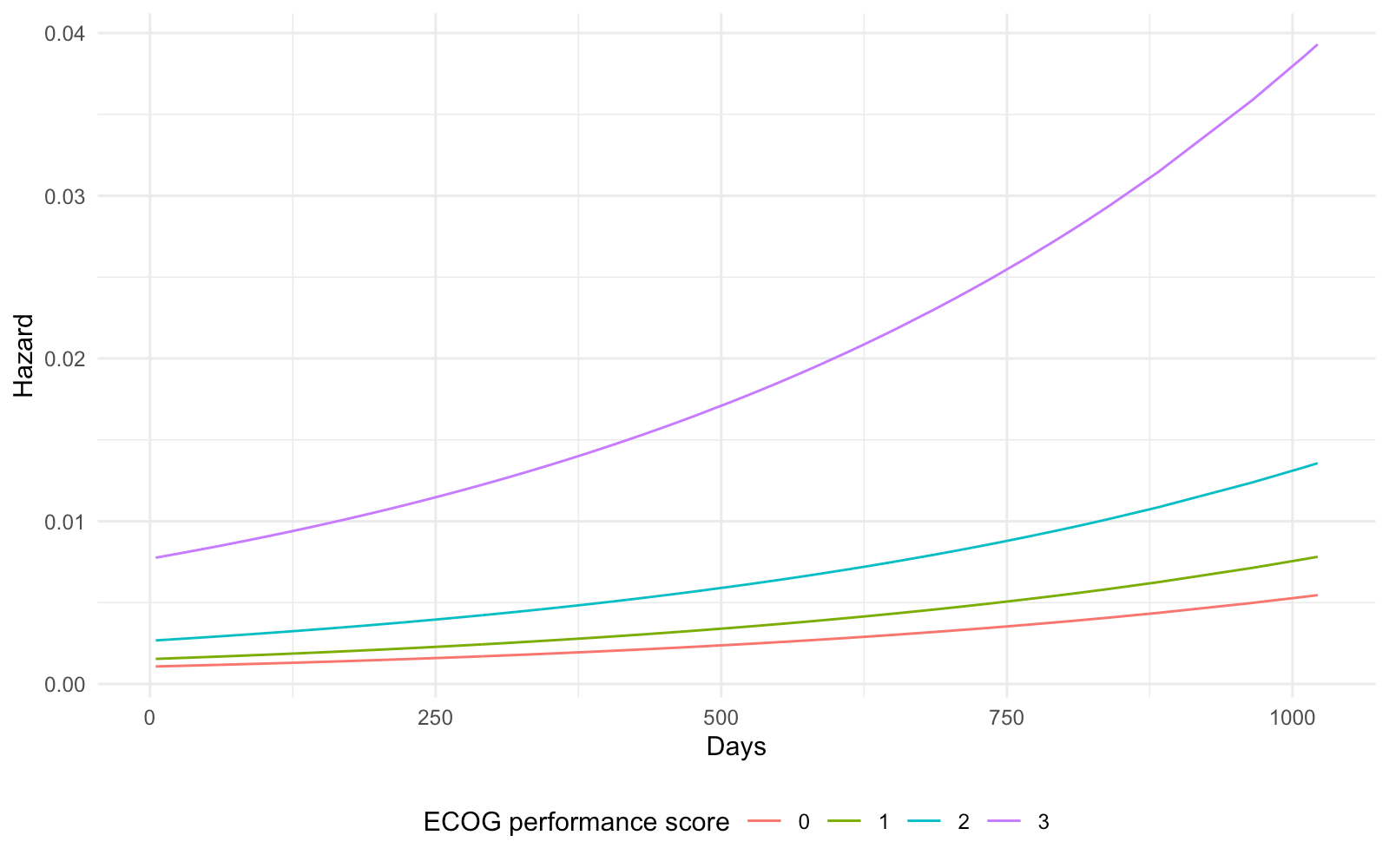# Conclusion

Parametric models are a useful technique for survival analysis, particularly when there is a need to extrapolate survival outcomes beyond the available follow-up data. R provides wide range of survival distributions and the flexsurv package provides excellent support for parametric modeling. Parametric distributions can support a wide range of hazard shapes including monotonically increasing, monotonically decreasing, arc-shaped, and bathtub-shaped hazards. However, in some cases, even the most flexible distributions such as the generalized gamma distribution may be insufficient. In these cases, flexible parametric models such as splines or fractional polynomials may be needed.